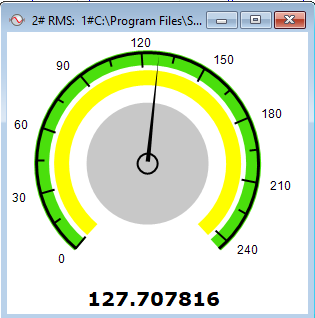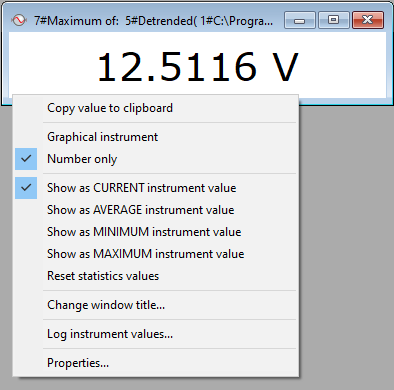To calculate and monitor the changes of some numeric value, you can use SIGVIEWs instruments. They calculate different values from the signals or 3D graphics and display them as a number or in graphical form as a round-scale instrument.

The right-click menu on the instrument window includes many useful options: copying instrument values to the clipboard, logging instrument values, editing instrument properties etc.

To automatically calculate and display the changes of an instrument value in a longer signal, you can also use Instrument values over time function.### Supported instrument types

You can create instruments by using one of the options in the “Instruments and markers” main menu section. SIGVIEW supports the following instrument types:

Value at (with marker)...:  You can define the X value from the signal and the instrument will monitor the Y value (for example, you can monitor FFT value on a certain frequency).

Marker with harmonics at...:  Available for FFT windows only. This shows value at desired frequency, along with markers on all harmonics of that frequency.

Mean: Calculates the mean value of the signal.

Sum: Calculates the sum of all signal values.

Const: Creates an instrument with a constant numerical value. This can be used for expressions in the Signal calculator. Const instrument value an be changed by using the corresponding context menu option "Change value...".

Const (child window with level marker): Same as Const instrument, except that it will be created as a child window of a signal window and will automatically display a level marker in the origin signal (see Instrument Properties for details about level markers).

Median value: Calculates median value from a signal. Displays a level marker in the signal window automatically (see Instrument Properties for details about level markers).

Quantile value...: Calculates Quantile value from a signal with arbitrary q-value. Displays level markers at quantile and max-quantile values in the signal window automatically (see Instrument Properties for details about level markers).

Fractal dimension...: Calculates Fractal Dimension of a signal by using Hurst Exponent method. It can be used to estimate a long-term memory of time series. An optimization level can be specified, defining a number of iterations for the "rescaled range optimization" algorithm (level of 1 is equal to 100000 iteration, level of 100 to 1000 iterations). Please note that the calculation of this value can be quite time-consuming. Therefore, a number of samples in a source signal is currently limited to 100000 samples. For longer input signals, this function will be disabled.

Standard deviation: Calculates the standard deviation of the visible part of the signal.

Variance: Measure of the amount of variation within the signal values, equal to the square of the standard deviation.

Skewness: Measure of symmetry, or more precisely, the lack of symmetry of distribution. A distribution, or data set, is symmetric if it looks the same to the left and right of the center point. The skewness for a normal distribution is zero, and any symmetric data should have a skewness near zero. Negative values for the skewness indicate data that are skewed left and positive values for the skewness indicate data that are skewed right.

Kurtosis: Measure of whether the data are peaked or flat, relative to a normal distribution. That is, data sets with high kurtosis tend to have a distinct peak near the mean, decline rather rapidly, and have heavy tails. Data sets with low kurtosis tend to have a flat top near the mean, rather than a sharp peak. A uniform distribution would be the extreme case. SIGVIEW uses "excess kurtosis", meaning that standard normal distribution has a kurtosis of zero.

RMS normalized: The same as the standard RMS function, but will first normalise the signal by subtracting its mean value from all signal values.

RMS (Root Mean Square): If the signal includes negative values, the sum instrument will probably not be useful. In that case you can use this instrument the square root of the mean of the squares of the signal values. RMS=sqrt((x12+x22+…..)/N).

RMS of a frequency range: Calculates normalized RMS limited to a defined frequency range. It is useful if you want to calculate how much energy is contained in a limited frequency range.

Overall dB (weighted): You can calculate overall sound pressure values weighted with standard A, C, or Z curves. This calculation is internally based on the 1/1 Octave plot values. These values are calculated in the background, weighted with the appropriate A, C or Z-weighting coefficients, and all bands are combined into one dB value.

Please note that the values calculated by using one of these functions may not match the values calculated by a sound pressure level meter. In order to achieve that, you would have to calibrate SIGVIEW first.

Crest factor: Calculates the crest factor of the visible part of the signal.

Integral: Calculates numeric integral over the visible signal part.

Signal-To-Noise Ratio (SNR): Calculates the ratio between signal power and noise power in the overall signal. The higher the ratio, the less obtrusive the background noise is. Since SIGVIEW does not have any information about the real nature of your signal, it will not be able to deliver accurate values in all cases. Therefore, you should use it with pure tone signals (i.e. sine) signals to achieve best accuracy. SNR units are decibel (dB).

Total Harmonic Distortion (THD) and Total Harmonic Distortion + Noise (THD+N): THD is a measurement of the harmonic distortion present in the signal and is defined as the ratio of the sum of the powers of all harmonic components to the power of the fundamental frequency. Lesser THD allows the components in a loudspeaker, amplifier or microphone, or other equipment, to produce a more accurate reproduction by reducing harmonics added by electronics and audio media. You should measure it in signals containing one pure tone (sine) component. THD+N means total harmonic distortion plus noise. This measurement is much more common and more comparable between devices. Both instrument values are calculate in decibel (dB). To convert dB values to percent values, you can use one of online calculators, for example here.

Weighted mean (Mean frequency): Calculates the sum of products xi * yi divided by the sum of yi (xi are values on x-axis and yi corresponding values on y-axis from origin plot). If applied to an FFT plot, it is equivalent to a mean frequency.

Weighted median (Median frequency): xi are values on the x-axis and yi corresponding values on the y-axis of the origin plot. This function calculates xi value so that the sum of xi * yi on both sides of the xi is the same (or nearly the same). If applied to an FFT plot, it is equivalent to a median frequency.

Maximum: Calculates maximum value from the signal.

Minimum: Calculates minimum value from the signal.

Maximum position: Calculates the position of maximum from the signal (for example, frequency of the max. peak in FFT).

Maximum position (interpolated): Estimates the position of the maximum value more precisely by using interpolation. It is especially useful for determining fundamental frequency in the spectrum with precision, better than the frequency resolution of the spectrum.

Minimum position: Calculates the position of minimum value from the signal.

Peak count: Shows the number of peaks detected by the peak detection function.

Zero-crossing rate: Average number of zero-crossing (positive to negative or vice versa) ins signal in one second

Peak-to-Peak: Distance between maximum and minimum values

Sum 3D over frequency range: This is the only instrument applicable to 3D graphics (usually Time FFT). It calculates the sum of all values from the 3D graphic for one segment of the Y-axis (usually frequency).

Show 5 highest peaks

The context menu for each signal window includes a “Show 5 highest peaks” option. With this option turned on, SIGVIEW will show 5 markers on the 5 highest signal values in the visible part of the signal.

To copy the values of all visible markers to the clipboard in a TAB-separated format, you can use the context menu option "Copy markers to clipboard" on the signal containing the markers.